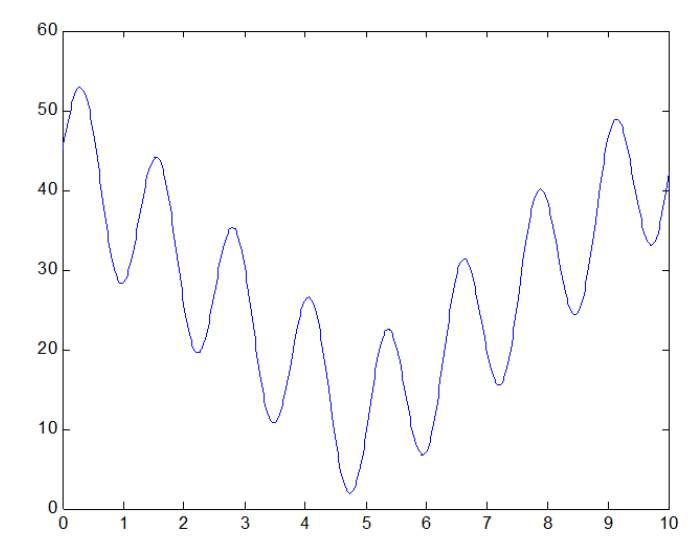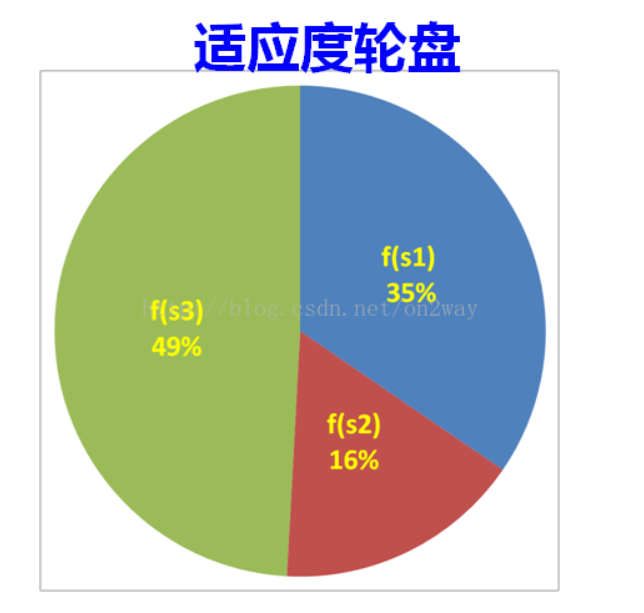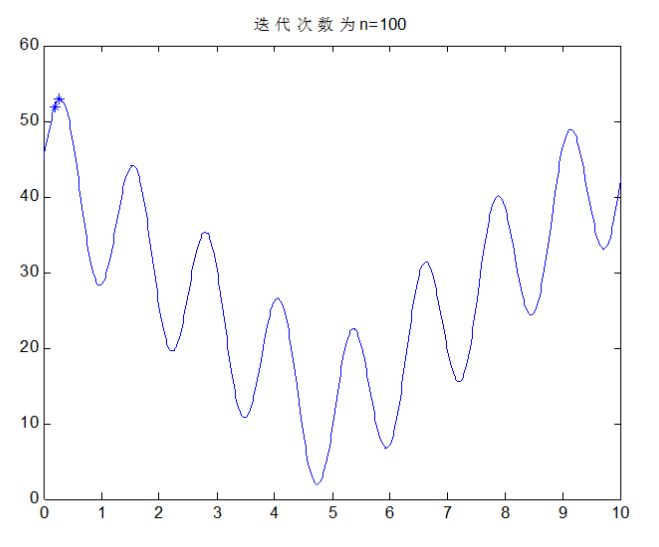# 看过最好的遗传算法和程序（没有之一）

1、遗传算法介绍

2、解决的问题3、如何开始？

4、编码

5、关于交叉与变异

6、关于选择的问题7、还差点什么呢（1）首先看主函数

function main()
clear;
clc;
%种群大小
popsize=100;
%二进制编码长度
chromlength=10;
%交叉概率
pc = 0.6;
%变异概率
pm = 0.001;
%初始种群
pop = initpop(popsize,chromlength);

for i = 1:100
%计算适应度值（函数值）
objvalue = cal_objvalue(pop);
fitvalue = objvalue;
%选择操作
newpop = selection(pop,fitvalue);
%交叉操作
newpop = crossover(newpop,pc);
%变异操作
newpop = mutation(newpop,pm);
%更新种群
pop = newpop;
%寻找最优解
[bestindividual,bestfit] = best(pop,fitvalue);
x2 = binary2decimal(bestindividual);
x1 = binary2decimal(newpop);
y1 = cal_objvalue(newpop);
if mod(i,10) == 0
figure;
fplot(‘10*sin(5*x)+7*abs(x-5)+10’,[0 10]);
hold on;
plot(x1,y1,’*’);
title([‘迭代次数为n=’ num2str(i)]);
%plot(x1,y1,’*’);
end
end
fprintf(‘The best X is —>>%5.2f\n’,x2);
fprintf(‘The best Y is —>>%5.2f\n’,bestfit);

（2）下面看二进制种群生成的方法

%初始化种群大小
%输入变量：
%popsize：种群大小
%chromlength：染色体长度–>>转化的二进制长度
%输出变量：
%pop：种群
function pop=initpop(popsize,chromlength)
pop = round(rand(popsize,chromlength));
%rand(3,4)生成3行4列的0-1之间的随机数
% rand(3,4)
%
% ans =
%
% 0.8147 0.9134 0.2785 0.9649
% 0.9058 0.6324 0.5469 0.1576
% 0.1270 0.0975 0.9575 0.9706
%round就是四舍五入
% round(rand(3,4))=
% 1 1 0 1
% 1 1 1 0
% 0 0 1 1
%所以返回的种群就是每行是一个个体，列数是染色体长度

（3）下面看如何把二进制返回对应的十进制

%二进制转化成十进制函数
%输入变量：
%二进制种群
%输出变量
%十进制数值
function pop2 = binary2decimal(pop)
[px,py]=size(pop);
for i = 1:py
pop1(:,i) = 2.^(py-i).*pop(:,i);
end
%sum(.,2)对行求和，得到列向量
temp = sum(pop1,2);
pop2 = temp*10/1023;

（4）下面看计算适应度函数：

%计算函数目标值
%输入变量：二进制数值
%输出变量：目标函数值
function [objvalue] = cal_objvalue(pop)
x = binary2decimal(pop);
%转化二进制数为x变量的变化域范围的数值
objvalue=10*sin(5*x)+7*abs(x-5)+10;

（5）如何选择新的个体

%如何选择新的个体
%输入变量：pop二进制种群，fitvalue：适应度值
%输出变量：newpop选择以后的二进制种群
function [newpop] = selection(pop,fitvalue)
%构造轮盘
[px,py] = size(pop);
totalfit = sum(fitvalue);
p_fitvalue = fitvalue/totalfit;
p_fitvalue = cumsum(p_fitvalue);%概率求和排序
ms = sort(rand(px,1));%从小到大排列
fitin = 1;
newin = 1;
while newin<=px
if(ms(newin))

11-2307-04
09-121万+
09-073万+
01-202642
11-063万+
05-103347
11-031万+
02-134972
12-195495
09-121万+
05-2910万+
01-051万+
06-22
03-241345
01-202万+
04-046504
12-265732
05-295820